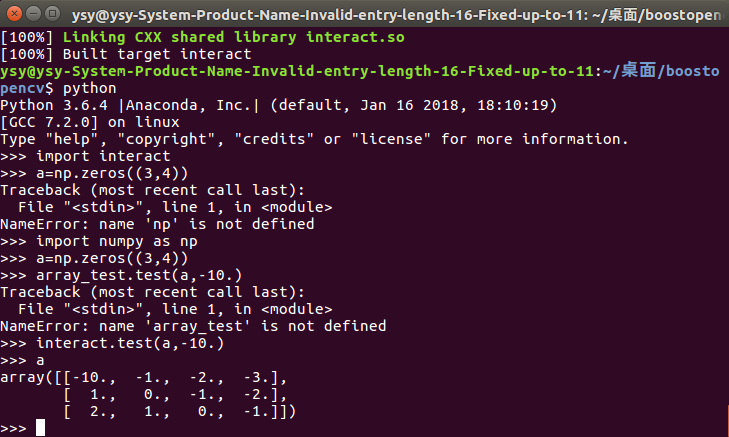## Boost.Python3将C++代码封装为Python模块，并在Python中调用封装的动态库so文件cmake， boost， opencv3，numpy_boost.python 封装so_little_YYYYY的博客-程序员宅基地

boost::python库是pyhon和c++相互交互的框架，可以在python中调用c++的类和函数等，也可以让c++调用python的类和方法。相比于ctype等，boost::python可以将C++代码更方便、快捷地移植到python模块当中，实现对python模块的扩充。

## opencv

c++函数中调用了opencv的库函数
interact.cpp

``````#include <stdio.h>
#include <opencv2/opencv.hpp>
#include <boost/python.hpp>

using namespace cv;

int main(){
}
void visualize()
{

cv::Mat image;
//cv::Mat GrayOriginal;
cvtColor(image, image, CV_BGR2GRAY);
image.convertTo(image, CV_64FC1, 1.0 / 255);
namedWindow("Display Image", WINDOW_AUTOSIZE );
imshow("Display Image", image);
waitKey(0);

// return image.at<cv::Vec3b>(2, 3);
}

using namespace boost::python;
BOOST_PYTHON_MODULE(interact) // file name
{

def("visualize", visualize); //function name
}
``````

CMakeLists.txt

``````cmake_minimum_required(VERSION 2.8)
project(interact)

FIND_PACKAGE(Boost COMPONENTS python)
FIND_PACKAGE(PythonInterp)
FIND_PACKAGE(PythonLibs)
include_directories(SYSTEM \${Boost_INCLUDE_DIR})
include_directories(/home/ysy/anaconda3/envs/ysy/include/python3.6m)

find_package( OpenCV REQUIRED )

set_target_properties(interact PROPERTIES PREFIX "")

``````

## python传numpy参数给c++函数

interact.cpp

``````#include<boost/python.hpp>
#include<boost/python/numeric.hpp>
#include<iostream>
using namespace boost::python;
void test_array(numeric::array &y, float value)
{

const tuple &shape= extract<tuple>(y.attr("shape"));
int h = extract<int>(shape);    // extract<int> numpy数据转为c++类型的数据
int w = extract<int>(shape);
for(int h_i=0;h_i<h;++h_i)
for(int w_i=0;w_i<w;++w_i)
y[make_tuple(h_i,w_i)]=h_i-w_i;
y[make_tuple(0,0)] = value;
}
BOOST_PYTHON_MODULE(array_test)
{

numeric::array::set_module_and_type("numpy", "ndarray");
def("test",&test_array);
}
``````

CMakeLists.txt

``````cmake_minimum_required(VERSION 2.8)
project(interact)

FIND_PACKAGE(Boost COMPONENTS python)

FIND_PACKAGE(PythonInterp)
FIND_PACKAGE(PythonLibs)
include_directories(SYSTEM \${Boost_INCLUDE_DIR})
include_directories(/home/ysy/anaconda3/envs/ysy/include/python3.6m)

set_target_properties(interact PROPERTIES PREFIX "")
``````参考这个
https://www.cnblogs.com/chenyliang/p/7474335.html

### BZOJ1013 [JSOI2008] 球形空间产生器sphere-程序员宅基地

Description　　有一个球形空间产生器能够在n维空间中产生一个坚硬的球体。现在，你被困在了这个n维球体中，你只知道球面上n+1个点的坐标，你需要以最快的速度确定这个n维球体的球心坐标，以便于摧毁这个球形空间产生器。Input　　第一行是一个整数n(1<=N=10)。接下来的n+1行，每行有n个实数，表示球面上一点的n维坐标。每一个实数精确到小数点后...

### python图像几何变换_Python实现图像几何变换-程序员宅基地

Python实现图像几何变换本文实例讲述了Python实现图像几何变换的方法。分享给大家供大家参考。具体实现方法如下：import Imagetry:im=Image.open('test.jpg')#out = im.resize((128, 128)) #改变大小#out = im.rotate(45) #45°旋转#out = im.transpose(Image.FLIP_LEFT_RIG...

### hjr-C++：VS2015+OpenCV3.1+Qt5-程序员宅基地

OpenCV安装程序常用数据结构QtQt+VS环境配置Qt结构OpenCVOpenCV是一个做图像处理的库，可以用C++，Java，Python来写图像处理的程序，比如图像滤波，变换，检测之类的，我们主要用vs2015+OpenCV3.1做图像处理。安装下载安装OpenCV，需要注意的是OpenCV版本要与vs版本对应，判断方法是\openc...

### web前端入门到实战：HTML-video标签_html video 预加载-程序员宅基地

​一、video标签1.作用：播放视频2.格式：<video src="视频地址“>3.可添加的属性：（1）autoplay="autoplay"代表可以在页面中自动播放，不可被用户中断（2）controls="controls”用于显示视频的控制条（3）poster="地址“用于播放视频之前显示什么东西（图片）（4）loop="loop"循环播放该视频（一般广告就是这么..._html video 预加载